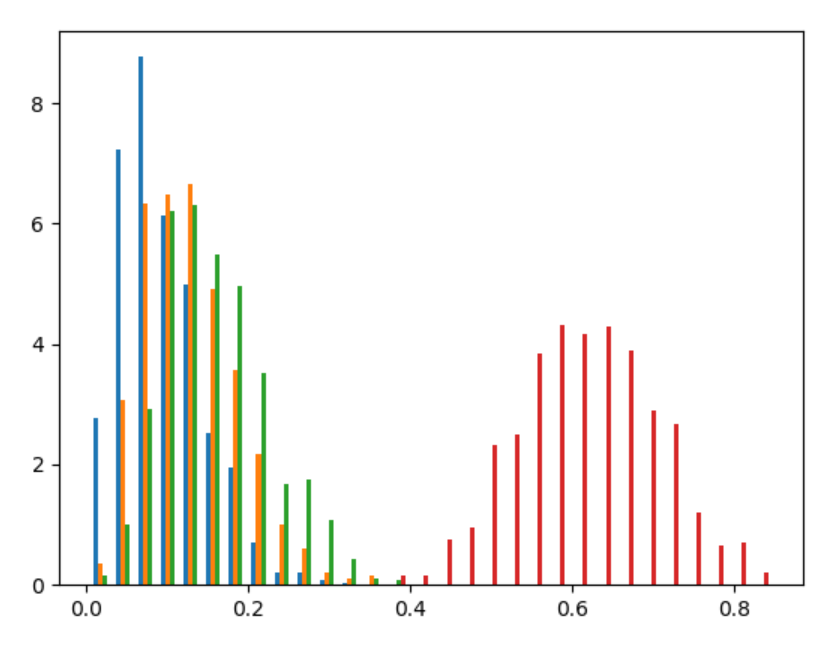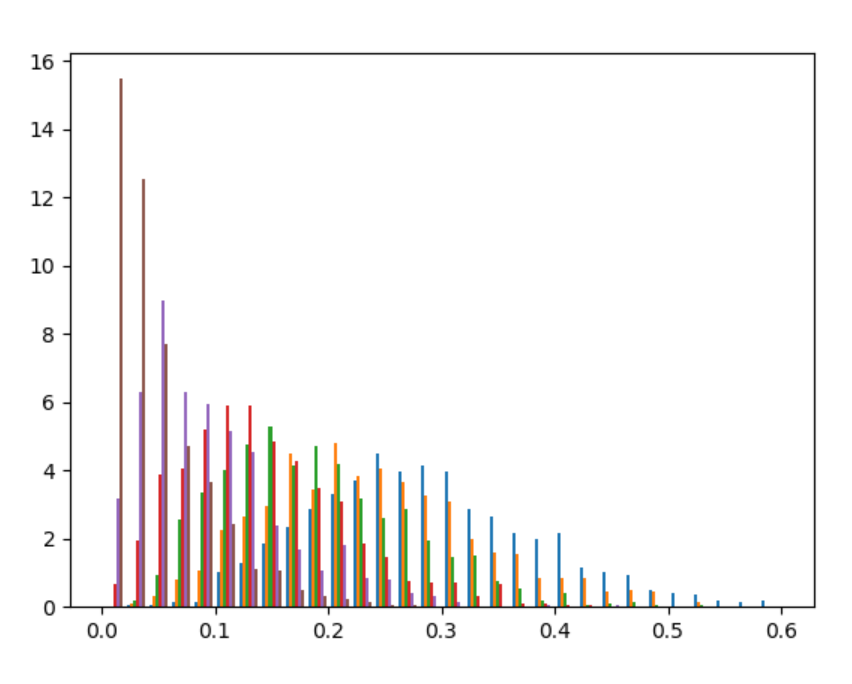Related Articles
numpy.random.dirichlet() in Python
• Last Updated : 15 Jul, 2020

With the help of dirichlet() method, we can get the random samples from dirichlet distribution and return the numpy array of some random samples by using this method.

Syntax : numpy.random.dirichlet(alpha, size=None)

Parameters :

1) alpha – number of samples.

2) size – output shape of a numpy array.

Return : Return the random samples array.

Example #1 :

In this example we can see that by using random.dirichlet() method, we are able to get the random samples of dirichlet distribution and return the numpy array having size defined in the parameters.

## Python3

 `# import dirichlet``import` `numpy as np``import` `matplotlib.pyplot as plt`` ` `# Using dirichlet() method``gfg ``=` `np.random.dirichlet((``3``, ``4``, ``5``, ``19``), size ``=` `1000``)`` ` `count, bins, ignored ``=` `plt.hist(gfg, ``30``, density ``=` `True``)``plt.show()`

Output :Example #2 :

## Python3

 `# import dirichlet``import` `numpy as np``import` `matplotlib.pyplot as plt`` ` `# Using dirichlet() method``gfg ``=` `np.random.dirichlet((``6``, ``5``, ``4``, ``3``, ``2``, ``1``), ``1000``)`` ` `count, bins, ignored ``=` `plt.hist(gfg, ``30``, density ``=` `True``)``plt.show()`

Output :Attention geek! Strengthen your foundations with the Python Programming Foundation Course and learn the basics.

To begin with, your interview preparations Enhance your Data Structures concepts with the Python DS Course.

My Personal Notes arrow_drop_up